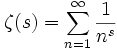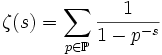# Riemann zeta-function

## Definition

### As a Dirichlet series

The Riemann zeta-function is the following Dirichlet series of:.

In other words, it is the Dirichlet series for the all ones function.

It can also be expressed as a product, using the product formula for Dirichlet series of completely multiplicative function:.

### As the function obtained by analytic continuation

The Dirichlet series for the Riemann zeta-function is absolutely convergent for all complex numbersfor which. Although the series does not make sense for other, the function extends to a meromorphic function of, with a single simple pole at the point.

### In terms of the Dirichlet eta-function

The Riemann zeta-function can be defined in terms of the Dirichlet eta-function:.

The Dirichlet eta-function is also defined in terms of a Dirichlet series, but this Dirichlet series has the advantage of being convergent on a wider range.

## Zeros and poles

### Poles

The Riemann zeta-function has a single simple pole at.

### Zeros

The Riemann zeta-function has zeros at all negative even integers. There are also infinitely many zeros with real part. The Riemann hypothesis states that all zeros other than the negative even integers have real part.